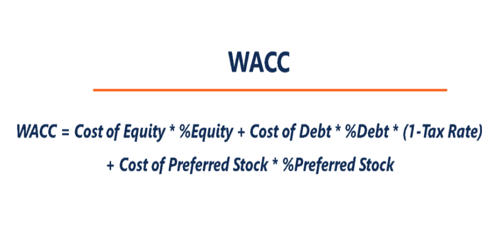Accounting

# Weighted Average Cost of Capital (WACC)Weighted Average Cost of Capital (WACC) is a calculation of a firm’s cost of capital in which each category of capital is proportionately weighted. Generally, projects are evaluated on the basis of the overall cost of capital, not on the basis of the specific cot of capital. All sources of capital, including common stock, preferred stock, bonds, and any other long-term debt, are included in a WACC calculation. As the majority of businesses run on borrowed funds, the cost of capital becomes an important parameter in assessing a firm’s potential for net profitability. The product of the component of cost of capital and weight respective source of capital is known as the weighted average cost of capital. Analysts and investors use the weighted average cost of capital (WACC) to assess an investor’s returns on an investment in a company.

In other words, the weighted average cost of capital is the minimum required rate of return to be earned on an investment. A firm’s WACC increases as the beta and rate of return on equity increase because an increase in WACC denotes a decrease in valuation and an increase in risk. Therefore, it is computed on the basis of the proportion of the funds from which the fund has been raised and their respective proportion.

A firm’s WACC represents its blended cost of capital across all sources, including common shares, preferred shares, and debt. Companies often run their business using the capital they raise through various sources. The cost of each type of capital is weighted by its percentage of total capital and they are added together. They include raising money by listing their shares on the stock exchange (equity), or by issuing interest-paying bonds or taking commercial loans (debt). All such capital comes at a cost, and the cost associated with each type varies for each source. This guide will provide a detailed breakdown of what WACC is, why it is used, how to calculate it, and will provide several examples.

Financial analysts use WACC widely in financial modeling as the discount rate when calculating the present value of a project or business. For example, a firm needs \$ 5,00,000 for investing in a new project. The firm can collect \$ 3,00,000 from shares on which it must pay a 12% dividend and \$ 2,00,000 from debentures on which it must pay 7% interest. If the fund is raised and invested in the project, the firm must earn at least \$ 50,000 which becomes sufficient to pay \$ 36,000 dividend(12% of 3,00,000) and \$ 14,000 interest (7% of 2,00,000) . The required earning (\$ 50,000) is 10% of the total fundraised. This 10% rate of return is called the weighted average cost of capital. The Weighted Average Cost of Capital shows us the relationship between the components of capital, commonly Equity and Debt.